Home > CC4 > Chapter 11 > Lesson 11.1.2 > Problem11-21

11-21.
1. Solve the following inequalities. Explain or justify your steps for part (a). Homework Help ✎

2.  a. 4x − 1 > 7 b. 3 − 2x < x + 6 c. 2(x − 5) < 8 d.x > 5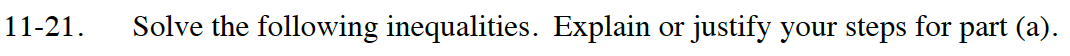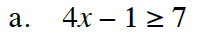Solve as an equation to find the boundary point.
Check points in each region to determine which region is the solution.

x ≥ 2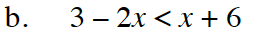Use the same method as part (a).

x > -1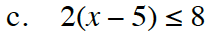Distribute before solving.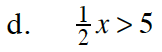Multiply the whole inequality by 2 to cancel out the fraction.